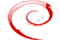Join the social network of Tech Nerds, increase skill rank, get work, manage projects...

• # Multiplication of integer values using shift operator (Binary multiplication)@kumar.abhishek• 0
• 1
• 0
• 0
• 0
• 0
• 0
• 0
• 294
Comment on it

There is an interesting way to multiply two positive integer values without using " * " operator and using binary shift operator. The complexity of the program will depends on the number of bits in the binary representation of the multiplier value.

The naive approach:

We can repeatedly add the value of multiplicand to a temporary variable, addition should be taken place until the addition operation is applied as the numerical value of the multiplier value. The time complexity of this program will depends on the value of multiplier O(n).

Sample program based on the above approach.

```#include <iostream>

using namespace std;

int main()
{
int a, b, i, tmp = 0;
cout << "Enter two integers to be multiplied : ";
cin >> a >> b;

for(i = 0; i < b; i++)
tmp += a;

cout << "Output " << a << " * " << b << " = " << tmp << endl;
return 0;
}
```

Output of the above program

```Enter two integers to be multiplied : 4 5
Output 4 * 5 = 20
```

A better approach:

Using the AND operator we are going to find the least significant bit in the multiplier by taking the AND operation with 1. If the LSB(left significant bit) of multiplier is 1 then we are going to add the value of multiplicand to a temporary variable, in case of 0 we skip the addition part. In each iteration we are applying left shift operation on multiplier and right shift operation on multiplicand by 1. When the value of multiplier is 0. We have the product of the integers with us. Time complexity of the above program will depends upon the number of bits in the binary representation of multiplier.

Sample program for the above approach

```#include <iostream>
#include <stack>

using namespace std;

int main()
{
int a, b, result = 0;
stack<int> st; //using stack for the proof of the program.

cout << "Enter two integers to be multiplied : ";
cin >> a >> b;
int tmp = 0;
int t1 = a;
int t2 = b;

while ( b != 0) {
if ( (b&1) == 1) {
result += a;
st.push(tmp);
}
tmp++;
a <<= 1;
b >>= 1;
}

cout << "Output " << t1 << " * " << t2 << " = " << result << endl;

//for printing the format in which shift has been applied..
//you can comment this part if you don't want it in your solution..
while(!st.empty()) {
cout << "(" << t1 << "<<" << st.top() << ")";
st.pop();
if (!st.empty()) {
cout << " + ";
}
}
cout << endl;

return 0;
}
```

Output of the above program

```Enter two integers to be multiplied : 4 5
Output 4 * 5 = 20
(4<<2) + (4<<0)
```

Corrections and suggestions are welcomed. :)

## 0 Comment(s)

OR
OR
Register

OR
Fill out the form below and instructions to reset your password will be emailed to you:

• Hire
• Post Projects

### Post Projects

• All at 0 Cost ....
• Post Tech Job
• Select Best Bidder
• Track the Project
• Approve Work and Pay safely
• Browse Nerds
• Work
• Find Projects Find Projects
• UI Design and UX
• Software Engineering
View more...
View less...
• Marketing
• General
• Manage
• Company Company

### Manage Company

• All at 0 Cost ....
• Manage Company and Employee Profiles
• Company wide Employee Productivity Reports
• Knowledge Sharing and Collaboration Tools
• Get Sales Lead and Bid for Tech Projects
• Send Invoices and Receive Payment Safely
• Learn
• Nerd Digest Nerd Digest
• UI Design and UX
• Software Engineering
View more...
View less...
• Marketing
• General
• Tech Q & A Tech Q & A
• UI Design and UX
• Software Engineering
View more...
View less...
• Marketing
• General